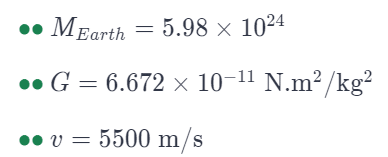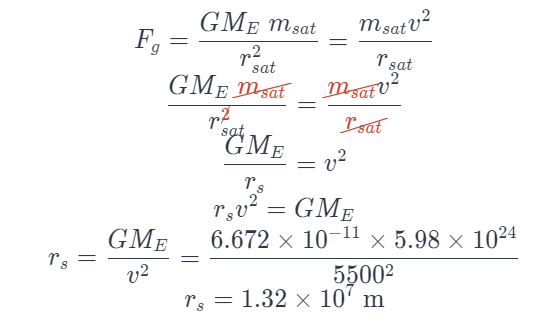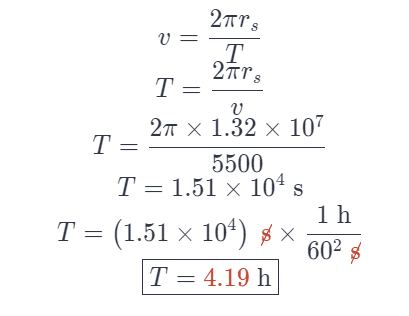# An Earth Satellite Moves In A Circular Orbit At A Speed Of 5500 m/s.

We thoroughly check each answer to a question to provide you with the most correct answers. Found a mistake? Let us know about it through the REPORT button at the bottom of the page.

An earth satellite moves in a circular orbit at a speed of 5500 m/s. What is its orbit period?

## Explanation

Givens:When a satellite is orbiting the Earth, the only force that is acting on it is the gravitational force between them.Notice that Rs is the radius of the satellite orbit.